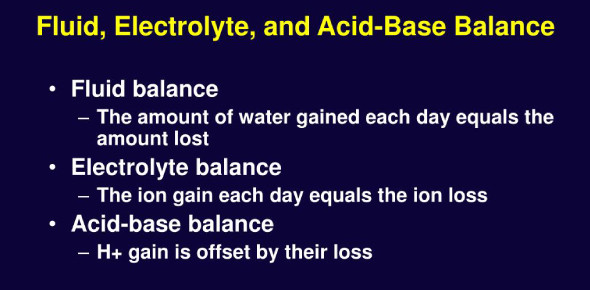# Trivia Quiz: Fluid, Electrolyte And Acid-base Balance!

34 Questions | Total Attempts: 2524SettingsCreate your own QuizAre you familiar with fluid, electrolyte, and acid-base balance? Do you think you can excel in this quiz? With this quiz, you will be accountable for knowing the two principle ions of extracellular fluid, four methods where body fluids and electrolytes move, what are two types of body fluids, and what body fluid is found outside cells. This quiz involves a basic understanding of fluids, electrolytes, and acid-base balance.

• 1.
Three compartments of ECF are:
• 2.
Examples of transcellular fluids are:
• 3.
Cite 4 examples of cations.
• 4.
Cite 4 examples of anions.
• 5.
What are the two principal ions of extracellular fluid?
• 6.
What are the two principal ions of intracellular fluid?
• 7.
Methods where body fluids and electrolytes move. Cite all 4.
• 8.
Rate of diffusion varies according to these three factors.
• 9.
Two types of body fluids.
• 10.
Also referred to as the cellular fluid. Is found within the cells of the body.
• 11.
Body fluid found outside the cells.
• 12.
Cite an example of intravascular fluid.
• 13.
Cite an example of interstitial fluid.
• 14.
It is the type of body fluid that acts as the transport system that carries nutrients to and waste products from the cells.
• 15.
This is a product of a gland and is considered as extracellular fluid.
• 16.
This is the waste produced by the cells of the body.
• 17.
How much percentage does fluid constitute in a healthy adult's weight?
• 18.
What is the percentage of fluid composition in infants?
• 19.
As people grow older, the fluid and fat proportions decrease.
• A.

True

• B.

False

• 20.
Body fat is essentially free of fluid.
• A.

True

• B.

False

• 21.
Women have proportionately more fat than men.
• A.

True

• B.

False

• 22.
Women have a smaller percentage of fluid in relation to total body weight than do men.
• A.

True

• B.

False

• 23.
When an excessive fluid loss occurs, ECF is the first volume to be depleted.
• A.

True

• B.

False

• 24.
Charged particles like Na+ are called electrolytes because they are capable of conducting electricity.
• A.

True

• B.

False

• 25.
Ions that carry positive charge are called...
Related TopicsBack to top
×

Wait!
Here's an interesting quiz for you.